# Selina Solutions Concise Mathematics Class 6 Chapter 19 Fundamental Operations Exercise 19(A)

Selina Solutions Concise Mathematics Class 6 Chapter 19 Fundamental Operations Exercise 19(A) provides answers in a step wise manner for better understanding of the concepts. The solutions help students in improving knowledge about the concepts, as per the current ICSE syllabus. Students can use these solutions, to get a new idea about the steps to be followed in solving the problems easily. Those who intend to speed up problem solving skills are advised to practice textbook questions using solutions designed by experts. Selina Solutions Concise Mathematics Class 6 Chapter 19 Fundamental Operations Exercise 19(A) PDF, links are given below for free download.

## Selina Solutions Concise Mathematics Class 6 Chapter 19: Fundamental Operations Exercise 19(A) Download PDF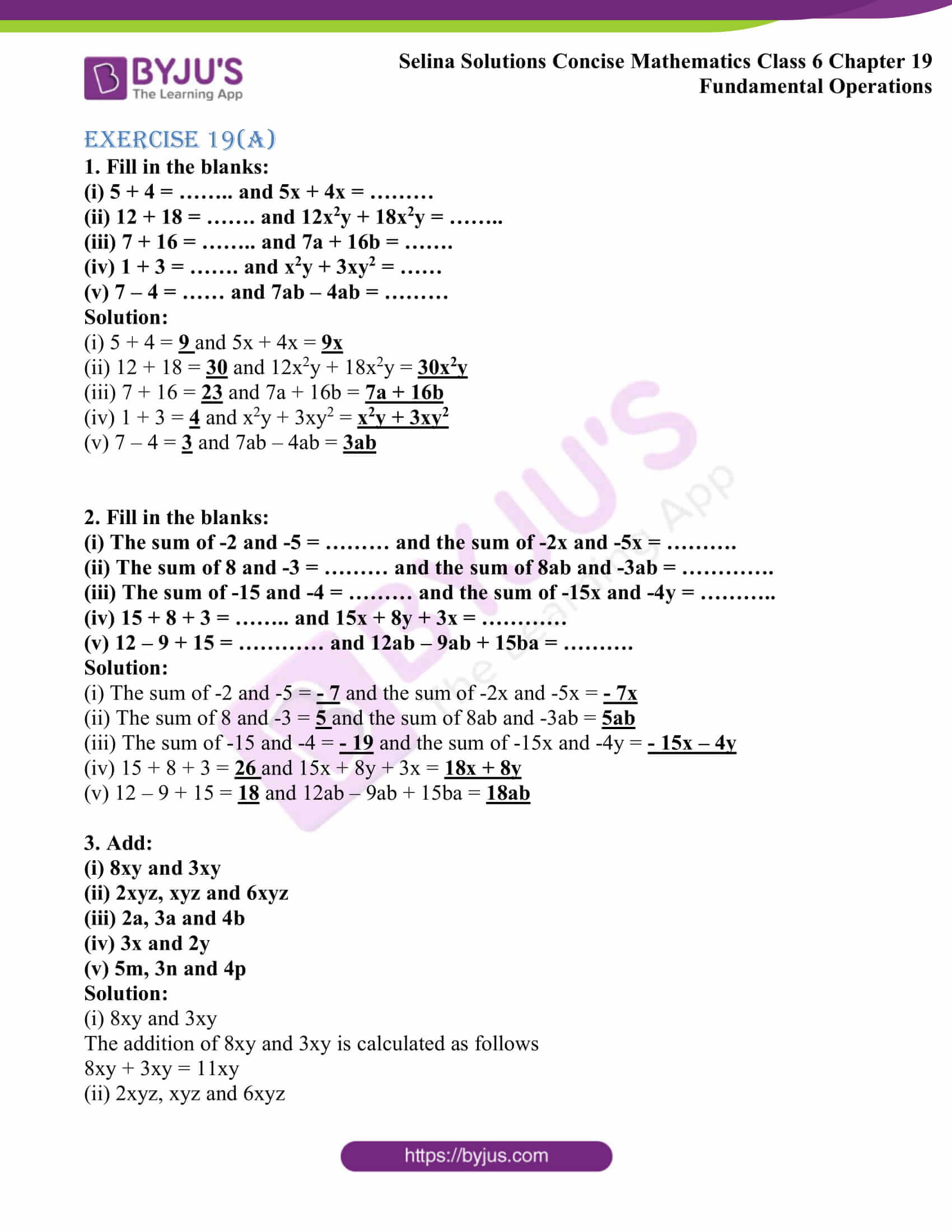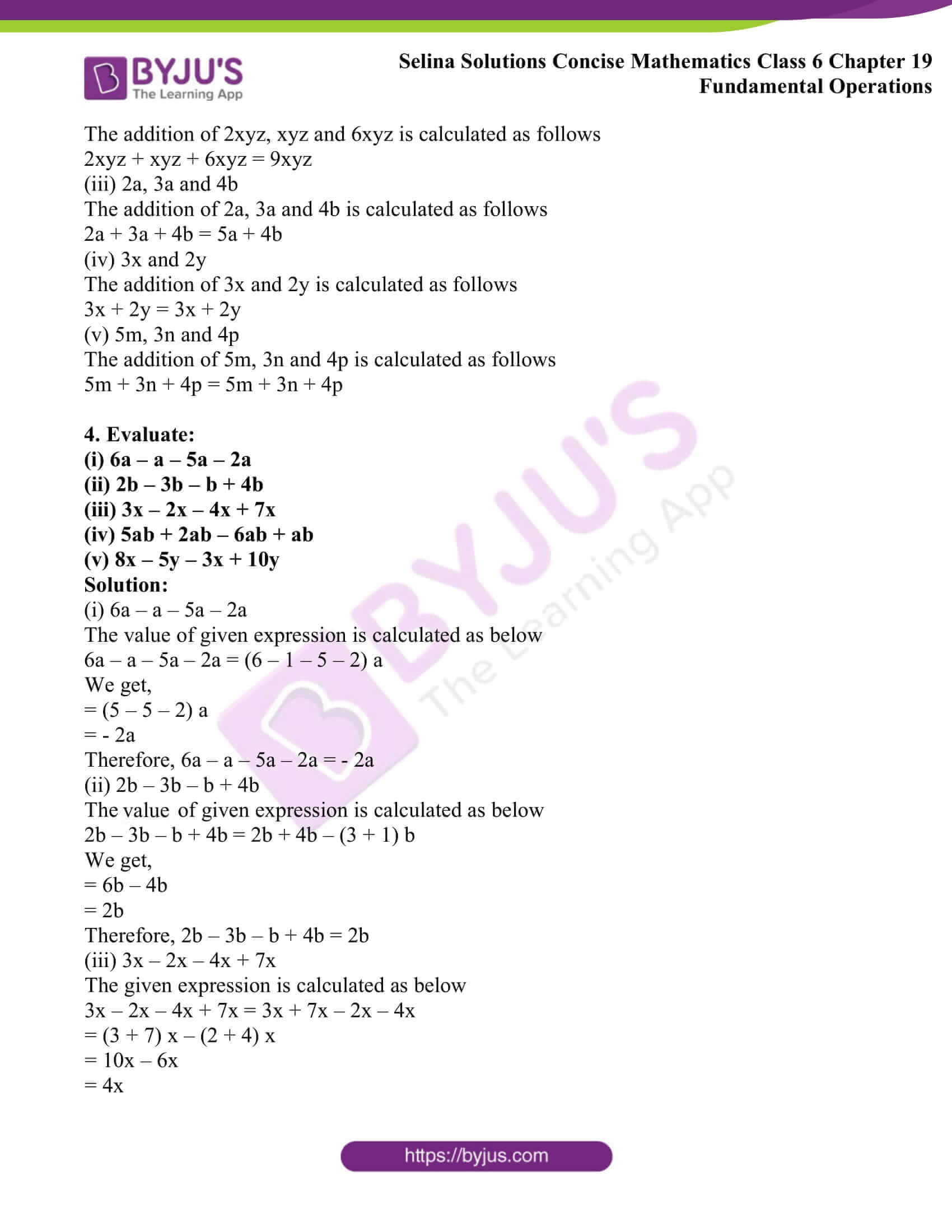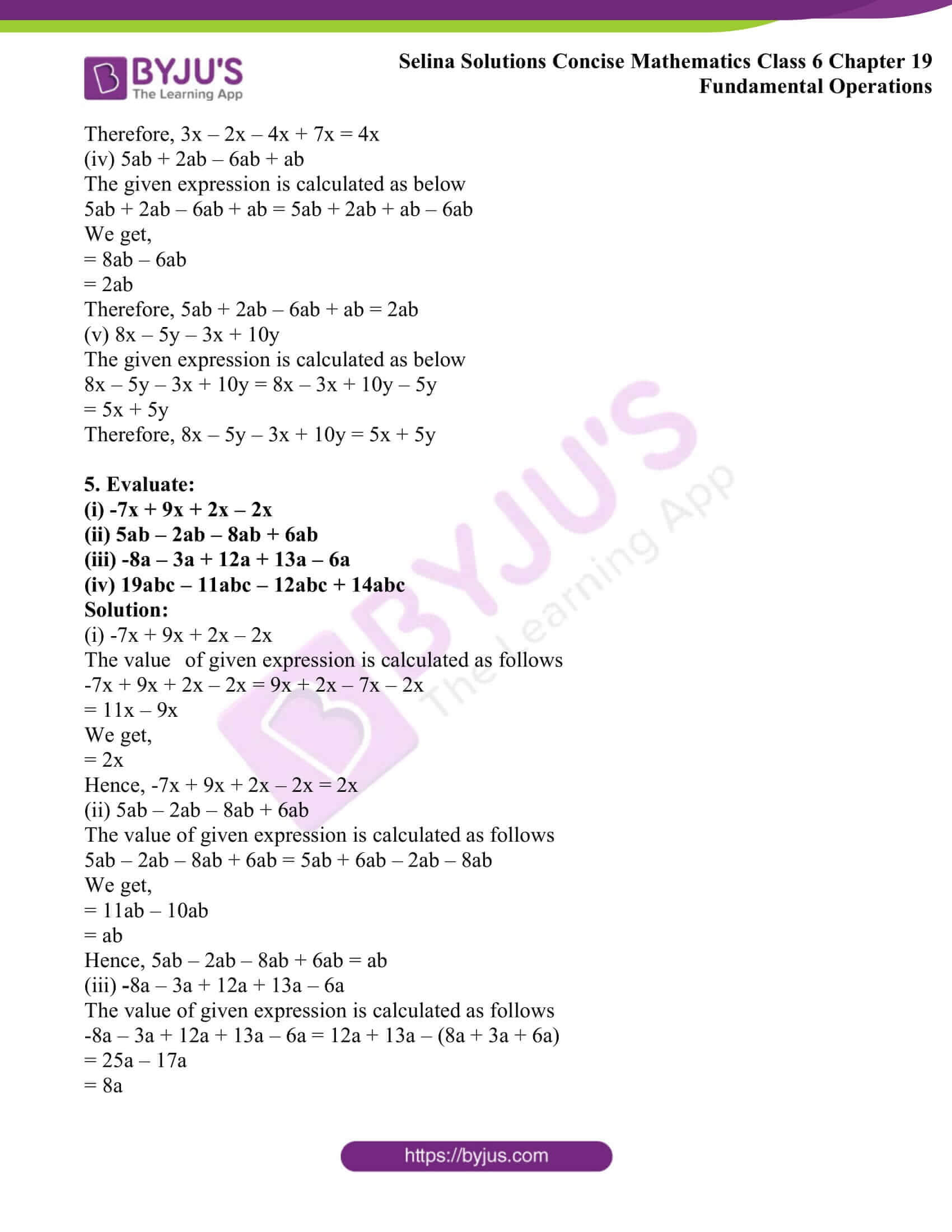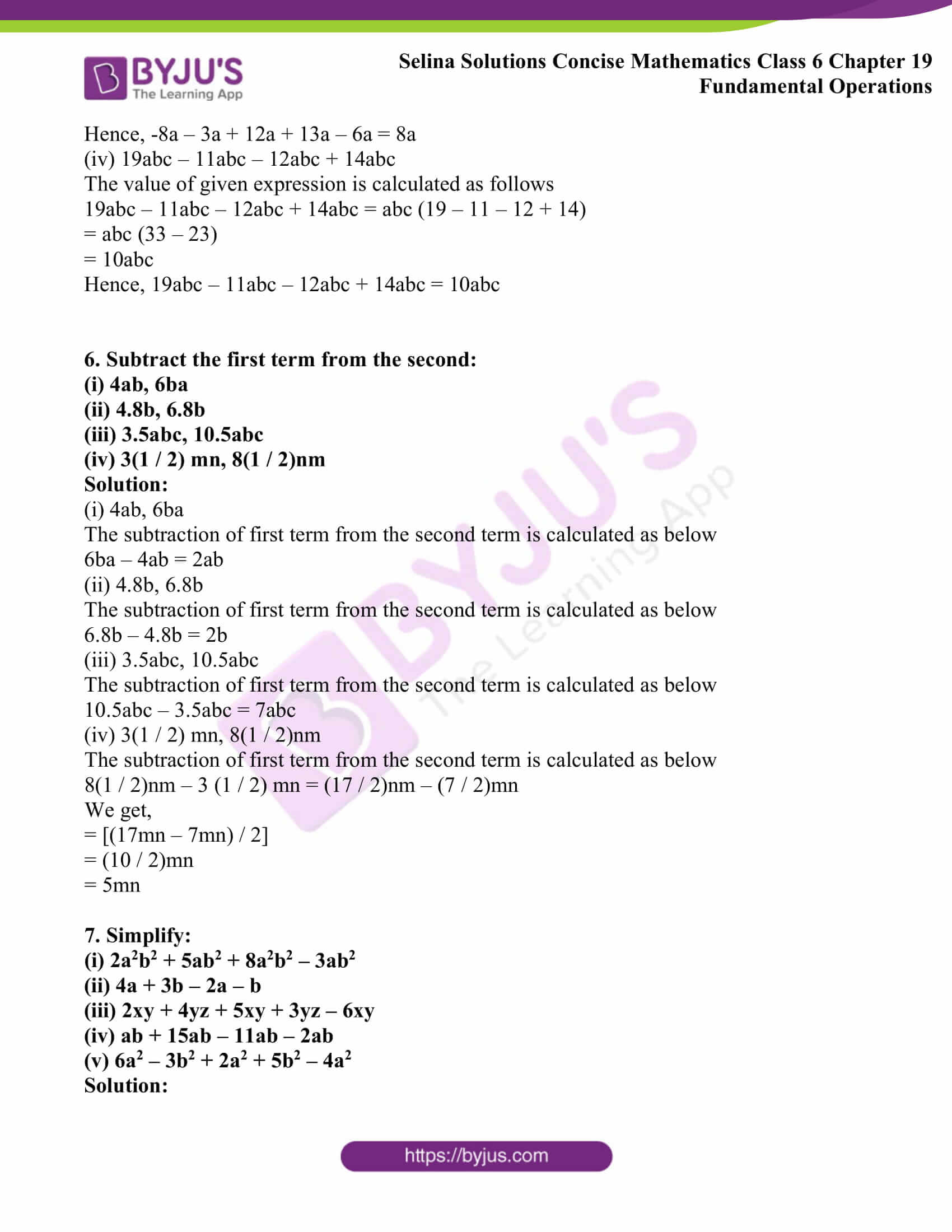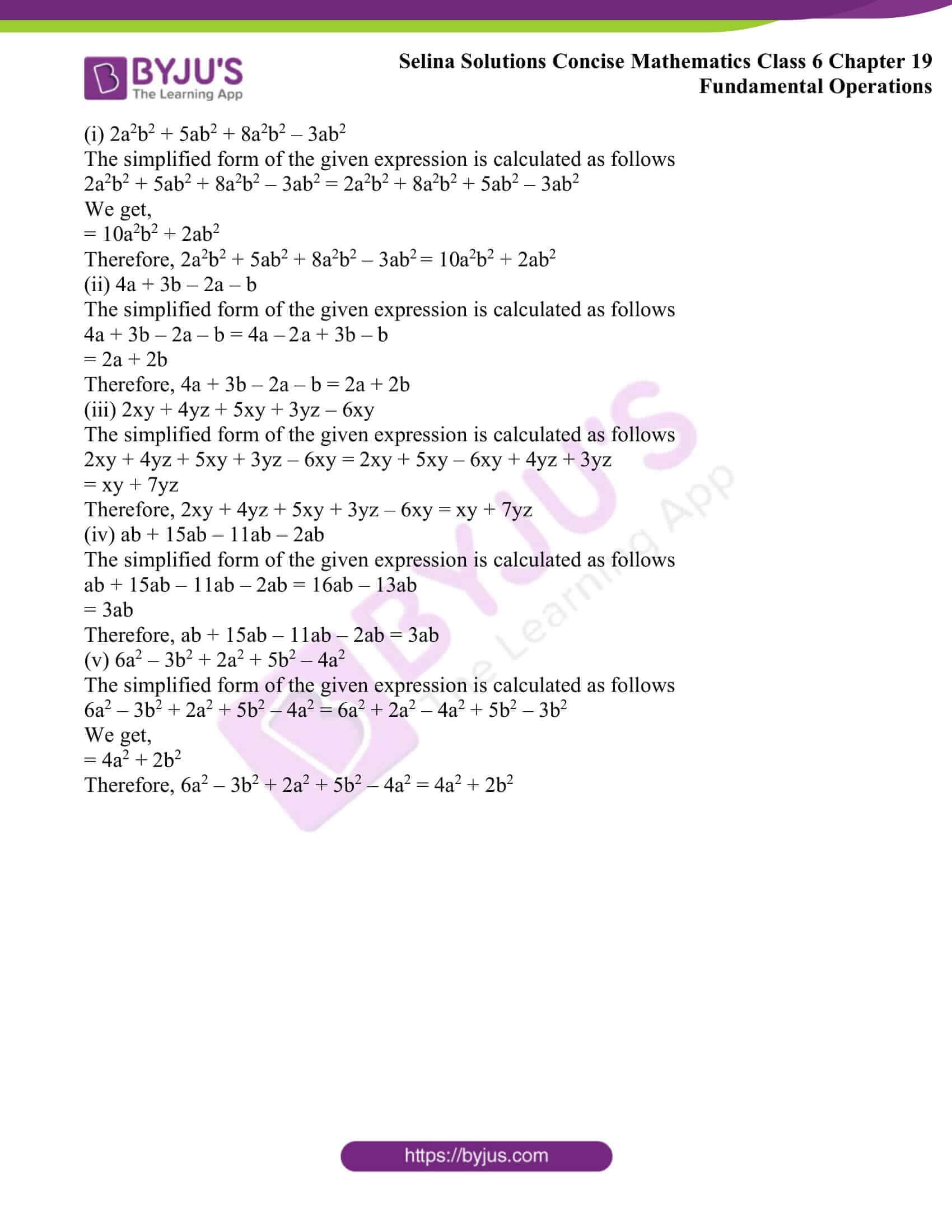### Access other exercises of Selina Solutions Concise Mathematics Class 6 Chapter 19: Fundamental Operations

Exercise 19(B) Solutions

Exercise 19(C) Solutions

Exercise 19(D) Solutions

### Access Selina Solutions Concise Mathematics Class 6 Chapter 19: Fundamental Operations Exercise 19(A)

Exercise 19(A)

1. Fill in the blanks:

(i) 5 + 4 = …….. and 5x + 4x = ………

(ii) 12 + 18 = ……. and 12x2y + 18x2y = ……..

(iii) 7 + 16 = …….. and 7a + 16b = …….

(iv) 1 + 3 = ……. and x2y + 3xy2 = ……

(v) 7 – 4 = …… and 7ab – 4ab = ………

Solution:

(i) 5 + 4 = 9 and 5x + 4x = 9x

(ii) 12 + 18 = 30 and 12x2y + 18x2y = 30x2y

(iii) 7 + 16 = 23 and 7a + 16b = 7a + 16b

(iv) 1 + 3 = 4 and x2y + 3xy2 = x2y + 3xy2

(v) 7 – 4 = 3 and 7ab – 4ab = 3ab

2. Fill in the blanks:

(i) The sum of -2 and -5 = ……… and the sum of -2x and -5x = ……….

(ii) The sum of 8 and -3 = ……… and the sum of 8ab and -3ab = ………….

(iii) The sum of -15 and -4 = ……… and the sum of -15x and -4y = ………..

(iv) 15 + 8 + 3 = …….. and 15x + 8y + 3x = …………

(v) 12 – 9 + 15 = ………… and 12ab – 9ab + 15ba = ……….

Solution:

(i) The sum of -2 and -5 = – 7 and the sum of -2x and -5x = – 7x

(ii) The sum of 8 and -3 = 5 and the sum of 8ab and -3ab = 5ab

(iii) The sum of -15 and -4 = – 19 and the sum of -15x and -4y = – 15x – 4y

(iv) 15 + 8 + 3 = 26 and 15x + 8y + 3x = 18x + 8y

(v) 12 – 9 + 15 = 18 and 12ab – 9ab + 15ba = 18ab

(i) 8xy and 3xy

(ii) 2xyz, xyz and 6xyz

(iii) 2a, 3a and 4b

(iv) 3x and 2y

(v) 5m, 3n and 4p

Solution:

(i) 8xy and 3xy

The addition of 8xy and 3xy is calculated as follows

8xy + 3xy = 11xy

(ii) 2xyz, xyz and 6xyz

The addition of 2xyz, xyz and 6xyz is calculated as follows

2xyz + xyz + 6xyz = 9xyz

(iii) 2a, 3a and 4b

The addition of 2a, 3a and 4b is calculated as follows

2a + 3a + 4b = 5a + 4b

(iv) 3x and 2y

The addition of 3x and 2y is calculated as follows

3x + 2y = 3x + 2y

(v) 5m, 3n and 4p

The addition of 5m, 3n and 4p is calculated as follows

5m + 3n + 4p = 5m + 3n + 4p

4. Evaluate:

(i) 6a – a – 5a – 2a

(ii) 2b – 3b – b + 4b

(iii) 3x – 2x – 4x + 7x

(iv) 5ab + 2ab – 6ab + ab

(v) 8x – 5y – 3x + 10y

Solution:

(i) 6a – a – 5a – 2a

The value of given expression is calculated as below

6a – a – 5a – 2a = (6 – 1 – 5 – 2) a

We get,

= (5 – 5 – 2) a

= – 2a

Therefore, 6a – a – 5a – 2a = – 2a

(ii) 2b – 3b – b + 4b

The value of given expression is calculated as below

2b – 3b – b + 4b = 2b + 4b – (3 + 1) b

We get,

= 6b – 4b

= 2b

Therefore, 2b – 3b – b + 4b = 2b

(iii) 3x – 2x – 4x + 7x

The given expression is calculated as below

3x – 2x – 4x + 7x = 3x + 7x – 2x – 4x

= (3 + 7) x – (2 + 4) x

= 10x – 6x

= 4x

Therefore, 3x – 2x – 4x + 7x = 4x

(iv) 5ab + 2ab – 6ab + ab

The given expression is calculated as below

5ab + 2ab – 6ab + ab = 5ab + 2ab + ab – 6ab

We get,

= 8ab – 6ab

= 2ab

Therefore, 5ab + 2ab – 6ab + ab = 2ab

(v) 8x – 5y – 3x + 10y

The given expression is calculated as below

8x – 5y – 3x + 10y = 8x – 3x + 10y – 5y

= 5x + 5y

Therefore, 8x – 5y – 3x + 10y = 5x + 5y

5. Evaluate:

(i) -7x + 9x + 2x – 2x

(ii) 5ab – 2ab – 8ab + 6ab

(iii) -8a – 3a + 12a + 13a – 6a

(iv) 19abc – 11abc – 12abc + 14abc

Solution:

(i) -7x + 9x + 2x – 2x

The value of given expression is calculated as follows

-7x + 9x + 2x – 2x = 9x + 2x – 7x – 2x

= 11x – 9x

We get,

= 2x

Hence, -7x + 9x + 2x – 2x = 2x

(ii) 5ab – 2ab – 8ab + 6ab

The value of given expression is calculated as follows

5ab – 2ab – 8ab + 6ab = 5ab + 6ab – 2ab – 8ab

We get,

= 11ab – 10ab

= ab

Hence, 5ab – 2ab – 8ab + 6ab = ab

(iii) 8a – 3a + 12a + 13a – 6a

The value of given expression is calculated as follows

-8a – 3a + 12a + 13a – 6a = 12a + 13a – (8a + 3a + 6a)

= 25a – 17a

= 8a

Hence, -8a – 3a + 12a + 13a – 6a = 8a

(iv) 19abc – 11abc – 12abc + 14abc

The value of given expression is calculated as follows

19abc – 11abc – 12abc + 14abc = abc (19 – 11 – 12 + 14)

= abc (33 – 23)

= 10abc

Hence, 19abc – 11abc – 12abc + 14abc = 10abc

6. Subtract the first term from the second:

(i) 4ab, 6ba

(ii) 4.8b, 6.8b

(iii) 3.5abc, 10.5abc

(iv) 3(1 / 2) mn, 8(1 / 2)nm

Solution:

(i) 4ab, 6ba

The subtraction of first term from the second term is calculated as below

6ba – 4ab = 2ab

(ii) 4.8b, 6.8b

The subtraction of first term from the second term is calculated as below

6.8b – 4.8b = 2b

(iii) 3.5abc, 10.5abc

The subtraction of first term from the second term is calculated as below

10.5abc – 3.5abc = 7abc

(iv) 3(1 / 2) mn, 8(1 / 2)nm

The subtraction of first term from the second term is calculated as below

8(1 / 2)nm – 3 (1 / 2) mn = (17 / 2)nm – (7 / 2)mn

We get,

= [(17mn – 7mn) / 2]

= (10 / 2)mn

= 5mn

7. Simplify:

(i) 2a2b2 + 5ab2 + 8a2b2 – 3ab2

(ii) 4a + 3b – 2a – b

(iii) 2xy + 4yz + 5xy + 3yz – 6xy

(iv) ab + 15ab – 11ab – 2ab

(v) 6a2 – 3b2 + 2a2 + 5b2 – 4a2

Solution:

(i) 2a2b2 + 5ab2 + 8a2b2 – 3ab2

The simplified form of the given expression is calculated as follows

2a2b2 + 5ab2 + 8a2b2 – 3ab2 = 2a2b2 + 8a2b2 + 5ab2 – 3ab2

We get,

= 10a2b2 + 2ab2

Therefore, 2a2b2 + 5ab2 + 8a2b2 – 3ab2 = 10a2b2 + 2ab2

(ii) 4a + 3b – 2a – b

The simplified form of the given expression is calculated as follows

4a + 3b – 2a – b = 4a – 2a + 3b – b

= 2a + 2b

Therefore, 4a + 3b – 2a – b = 2a + 2b

(iii) 2xy + 4yz + 5xy + 3yz – 6xy

The simplified form of the given expression is calculated as follows

2xy + 4yz + 5xy + 3yz – 6xy = 2xy + 5xy – 6xy + 4yz + 3yz

= xy + 7yz

Therefore, 2xy + 4yz + 5xy + 3yz – 6xy = xy + 7yz

(iv) ab + 15ab – 11ab – 2ab

The simplified form of the given expression is calculated as follows

ab + 15ab – 11ab – 2ab = 16ab – 13ab

= 3ab

Therefore, ab + 15ab – 11ab – 2ab = 3ab

(v) 6a2 – 3b2 + 2a2 + 5b2 – 4a2

The simplified form of the given expression is calculated as follows

6a2 – 3b2 + 2a2 + 5b2 – 4a2 = 6a2 + 2a2 – 4a2 + 5b2 – 3b2

We get,

= 4a2 + 2b2

Therefore, 6a2 – 3b2 + 2a2 + 5b2 – 4a2 = 4a2 + 2b2